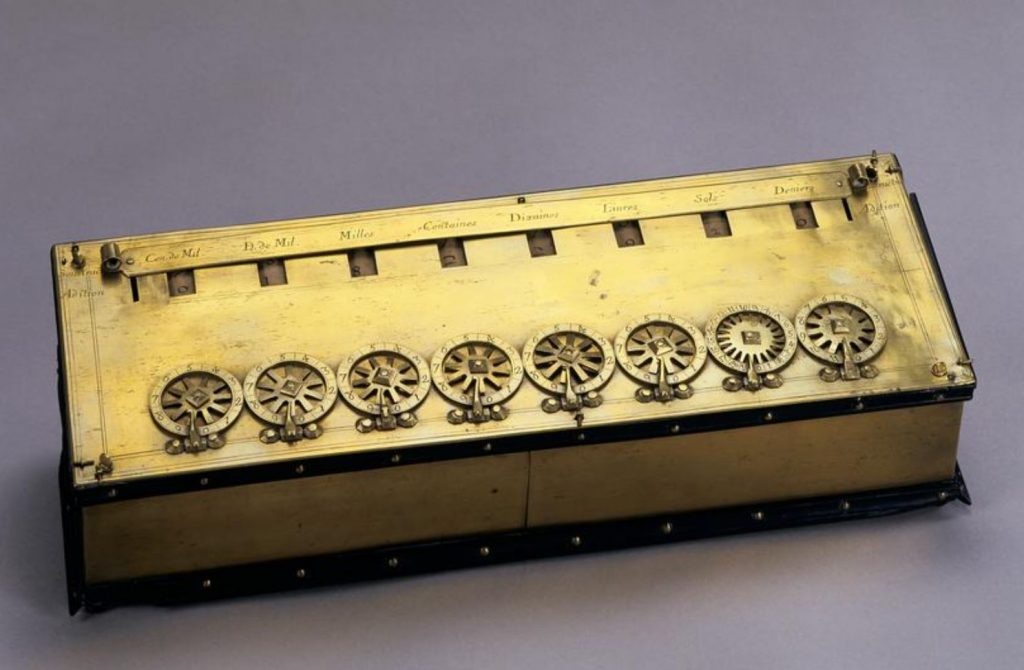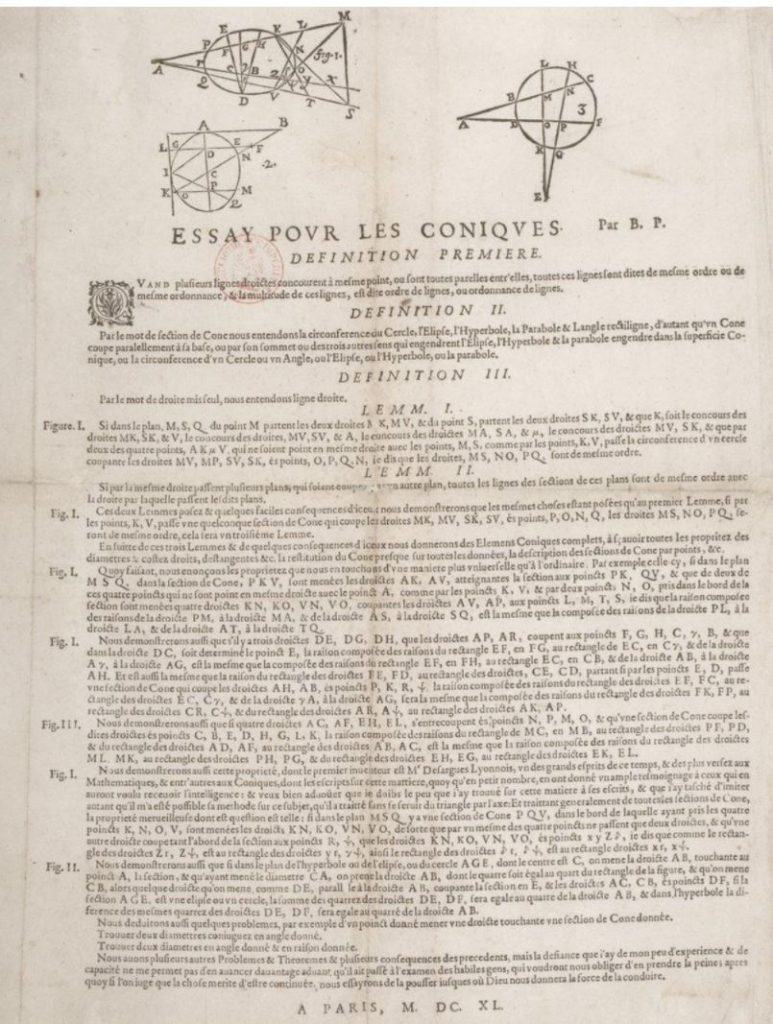# Probability, statistics and Pascal’s other contributions

This is the final part in the Pascal pentalogy, a series of guest posts by David Benjamin exploring the secrets of Pascal’s Triangle.

## Probability and combinations

In Part 1 of this series we stated that Pascal is credited with being the founder of probability theory – but credit also needs to be given to other mathematicians, in particular the Italian polymath Girolamo Cardano.

The connection between probability and the numbers in Pascal’s triangle can be shown by looking at the outcomes when one or more coins are tossed. The table below, from row two, lists the outcomes for one, two and three unbiased coins.

For four coins there is $1$ outcome for four heads, $4$ outcomes for three heads and one tail, $6$ outcomes for two heads and two tails, $4$ outcomes for one head and three tails and one outcome for $4$ tails.

Row four shows us that when three unbiased coins are tossed, the probability they will land showing two heads and one tail in any order is $\frac{3}{1+3+3+1}=\frac{3}{8}$.

As the sum of the $n^{th}$ row is $2^{n}$, the number of possible outcomes for four coins is $2^4=16$, $32$ for five coins, $64$ for six coins, …

## Quincunx

A Quincunx, or Galton Board, is named after the English explorer and anthropologist Francis Galton (1822-1911) – although this name is now less popular, because of Galton’s views on eugenics and racist attitudes.

The board is a triangular array of pegs. Balls are dropped onto the top peg and then bounce their way down to the bottom where they are collected in containers. Each time a ball hits one of the pegs, it bounces either left or right with an equal probability of $\frac{1}{2}$ and the balls collect in the containers to form the classic bell-shaped curve of the normal distribution.

The Quincunx is like Pascal’s triangle with pegs instead of numbers. The number on each peg represents the number of different paths a ball can take to reach that peg. If there are $10$ rows and the last row contains the containers, then the probability of landing in the third container from the right can be calculated by using the formula for the Binomial distribution.

The probability of landing in the third bin from the right is $120\times(\frac{1}{2})^3\times(\frac{1}{2})^7=\frac{15}{128}=0.1171875$, where $120$ is the number of different paths to that bin.

## Statistics and permutations

The link between statistics and the triangle can be demonstrated using combinations. Consider these 5 mathematicians Euler, Pascal, Ramanujan, Hilbert and Conway and the possible teams for a three-legged race.

There are $10$ different teams of $3$:

EPR      EPH     EPC     ERH    ERC   EHC    PRH    PRC    PHC    RHC

The formula to calculate the number of combinations is $_n{C}_r =\frac{n!}{r!(n-r)!}$ where $n$ represents the total we are choosing from, $r$ the number in the team and

$n!=n\times(n-1)\times(n-2)\times(n-3)\times…\times1$

In our example $n=5$, $r=3$ and $\frac{5!}{3!(5-3)!}=\frac{120}{6\times2}=10$

$_n{C}_r$ can be used to calculate the rows of Pascal’s triangle as shown below for row $6$, where in the calculation of $_5{C}_0$, $0!=1$

The animation film Of Dice and Men by John Weldon is a lovely way to introduce students to probability and statistics.

## Pascal the polymath: mathematics, inventor, science and religion

Pascal’s father was a tax collector and in 1642 Blaise invented a mechanical calculator to assist his father. It was called the Pascaline and had a wheel with eight movable parts for dialing. Each part corresponded to a particular digit in a number. Numbers could be added by turning the wheels located along the bottom of the machine. Subtraction was carried out by exploiting a method called nines’ complement representation, the use of which allows subtraction to be reduced to addition. Each digit in the answer was displayed in a separate window. The workings of the Pascaline are demonstrated here.A Pascaline. An original is displayed in The Musée des Arts et Métiers in Paris, France

The Musée des Arts et Métiers in Paris has one of the original Pascalines. The invention was not a commercial success – it was very expensive and often only purchased as a novelty rather than for use. Essentially, it was an adding machine. Subtraction was turned into a form of addition, as was multiplication. Division was done by repeated subtraction. Nines’ complement representation is still used in modern digital computers by a similar technique called ones’ complement which is used to represent negative numbers and hence perform subtraction in the same way as addition. Pascal did not discover this method but his calculator is the earliest known device to employ it. He continued to make improvements to his design until 1652.

Conic sections – normally just called conics – are obtained when a mathematical cone is sliced by a plane. Depending on the angle of the slice, the intersections create a circle, an ellipse, a parabola and a hyperbola. Conics have many applications including the wheel of course, ophthalmic, parabolic mirrors and reflectors, telescopes, searchlights and projectile motion.

Pascal wrote a short treatise, Essai pour les coniques (Essay on Conics) when only 16. In it he included what is known as Pascal’s Theorem which states that if a hexagon is inscribed in a conic section then the three intersection points of opposite sides lie on a straight line – the Pascal line. The theorem [also referred to as Pascal’s Hexagrammum Mysticum Theorem] was his first important mathematical discovery and a breakthrough in the field of projective geometry.A rare copy of the Essay pour les coniques which is kept in the National Library of France

In 1647 Pascal expanded on the work of the Italian physicist Evangelista Torricelli, the inventor of the barometer by writing Experiences nouvelles touchant le vide (New experiments with the vacuum) in which Pascal gave detailed rules to describe to what degree various liquids could be supported by air pressure. In 1971 the SI unit for pressure [equal to one newton per square metre] was named the pascal.

Also in 1647 he discovered Pascal’s Law of hydrostatics allowing for the development of the hydraulic press. Pascal himself used the principle to invent the syringe.

Pascal wrote an extremely influential theological work which was unfinished at the time of his death. It was posthumously called Pensées (Thoughts) and contained a detailed and coherent examination and defence of the Christian faith.

In 1655 Pascal was trying to invent a perpetual motion machine, a machine that continues to operate without drawing energy from an external source. The laws of physics now say this is impossible. Naturally he failed but he ended up inventing a basic roulette wheel, now upgraded and used in casinos as a game of chance.

The Swiss computer scientist Niklaus Emil Wirth, born in 1934, named one of his programming languages Pascal in honour of Blaise. Wirth along with Helmut Weber also designed the programming language named after another mathematician, Euler. [Recommended read: Euler: The Master of Us All ]

Pascal died in extreme pain at the young age of 39. He had a malignant growth in his stomach which had spread to his brain. Like many others, such as Évariste Galois and Franz Schubert, we are left wondering what else Pascal could have achieved had he lived longer. His work with Fermat into the calculus of probabilities helped the German mathematician Gottfried Leibniz [1646-1716] develop the infinitesimal calculus. Pascal is buried in the Saint-Étienne-du-Mont church in Paris and his death mask is held at the J. Paul Getty museum in Los Angeles, California.

•#### David Benjamin

Teacher of mathematics [1975-2018], Author [Heinemann, Stanley Thornes], Key contributor teachitmaths, Mathematics software producer [Virtual Image]

### 4 Responses to “Probability, statistics and Pascal’s other contributions”

1.Anna

Great series!

2.Stuart Goodall